# Telling the Time: Using 24-Hour Clocks

In this worksheet, students read an analogue clock face and give the time in 24-hour clock format.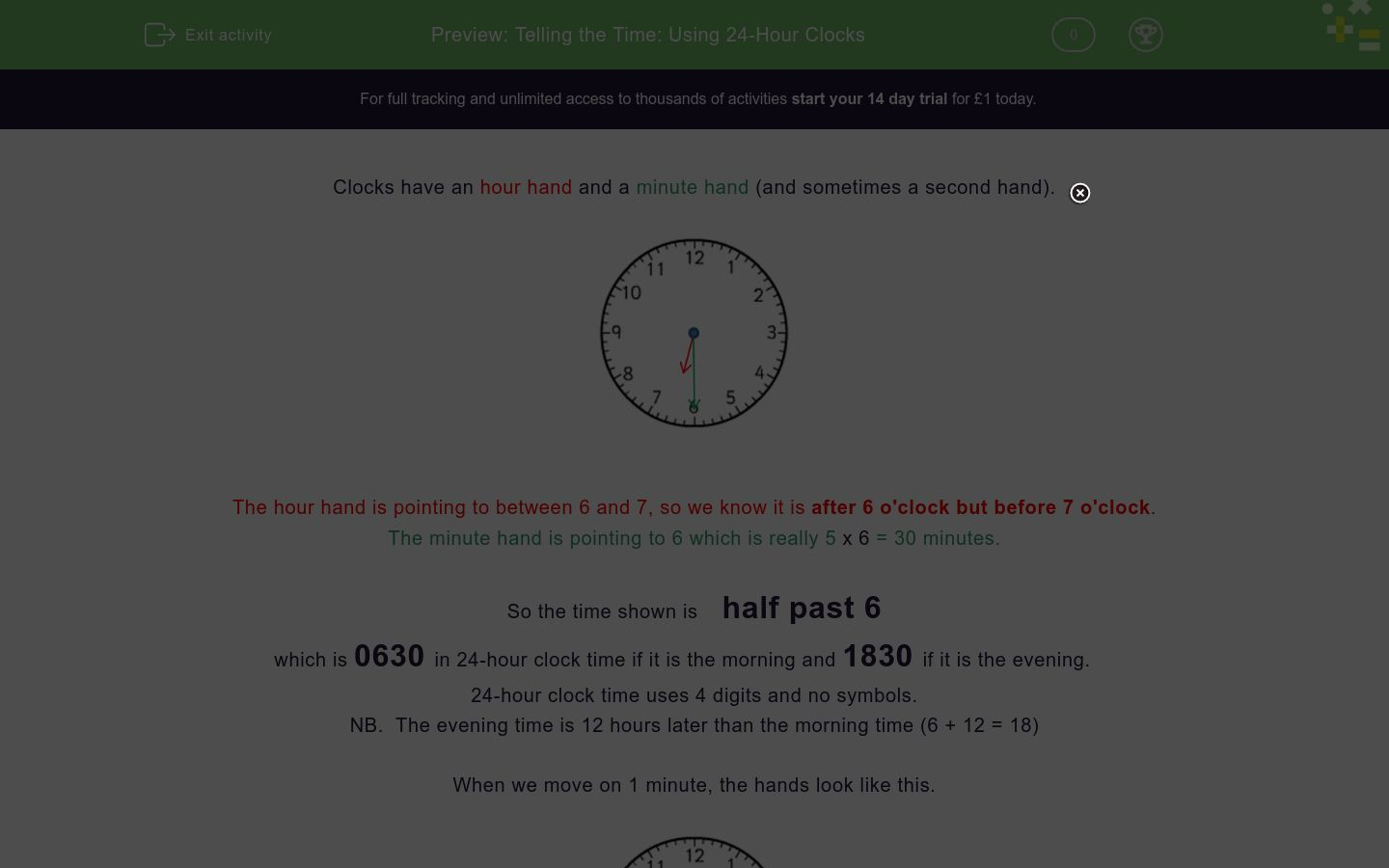Key stage:  KS 2

Curriculum topic:   Measurement

Curriculum subtopic:   Convert Analogue/Digital Time

Difficulty level:### QUESTION 1 of 10

Clocks have an hour hand and a minute hand (and sometimes a second hand).The hour hand is pointing to between 6 and 7, so we know it is after 6 o'clock but before 7 o'clock.

The minute hand is pointing to 6 which is really 5 x 6 = 30 minutes.

So the time shown is    half past 6

which is 0630 in 24-hour clock time if it is the morning and 1830 if it is the evening.

24-hour clock time uses 4 digits and no symbols.

NB.  The evening time is 12 hours later than the morning time (6 + 12 = 18)

When we move on 1 minute, the hands look like this.The hour hand has moved a tiny tiny tiny amount.

The minute hand has moved forward one minute.

So the time shown is    0631   or         1831

Want to understand this further and learn how this links to other topics in maths?
Why not watch this video?

What is the exact time in the morning, shown on this clock face?6:31

0629

629

What is the exact time, shown on this clock face in the morning?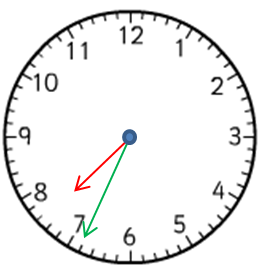0707

0734

0708

What is the exact time, shown on this clock face in the morning?What is the exact time, shown on this clock face in the evening?2117

0917

917

What is the exact time, shown on this clock face at night?2327

1127

1756

What is the exact time, shown on this clock face at night?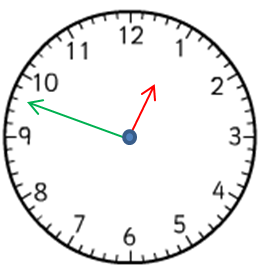1209½

0048

1248

What is the exact time, shown on this clock face in the afternoon?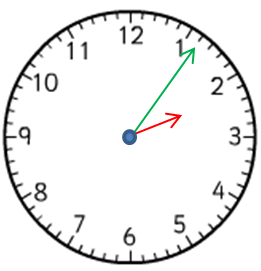1406

0206

1311

What is the exact time, shown on this clock face at night?1012½

2203

1250

What is the exact time, shown on this clock face at tea time?What is the exact time, shown on this clock face early in the morning?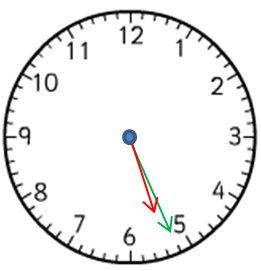• Question 1

What is the exact time in the morning, shown on this clock face?0629
EDDIE SAYS
The hour hand is past the 6 and the minute hand is on 29. On the 24-hour clock this would be 0629.
• Question 2

What is the exact time, shown on this clock face in the morning?0734
EDDIE SAYS
The hour hand is past the 7 and the minute hand is on 34. This would be 0734 on the 24-hour clock.
• Question 3

What is the exact time, shown on this clock face in the morning?0856
EDDIE SAYS
The hour hand is past the 8 and the minute hand is on 56, so 0856 on the 24-hour clock.
• Question 4

What is the exact time, shown on this clock face in the evening?2117
EDDIE SAYS
The hour hand is past the 9 and the minute hand is on 17. On the 24-hour clock this would be 2117.
• Question 5

What is the exact time, shown on this clock face at night?2327
EDDIE SAYS
The hour hand is past the 11 and the minute hand is on 27. On the 24-hour clock this would be 2327.
• Question 6

What is the exact time, shown on this clock face at night?0048
EDDIE SAYS
The hour hand is past the 12 and the minute hand is on 48. On the 24-hour clock this would be 0048.
• Question 7

What is the exact time, shown on this clock face in the afternoon?1406
EDDIE SAYS
The hour hand is past the 2 and the minute hand is on 6. On the 24-hour clock this would be 1406.
• Question 8

What is the exact time, shown on this clock face at night?2203
EDDIE SAYS
The hour hand is on the 10 and the minute hand is on the 3. On the 24-hour clock this would be 2203.
• Question 9

What is the exact time, shown on this clock face at tea time?1613
EDDIE SAYS
The hour hand is past the 4 and the minute hand is on 13. On the 24-hour clock it would be 1613.
• Question 10

What is the exact time, shown on this clock face early in the morning?0526
EDDIE SAYS
The hour hand is past the 5 and the minute hand is on 26, or 0526.
---- OR ----

Sign up for a £1 trial so you can track and measure your child's progress on this activity.

### What is EdPlace?

We're your National Curriculum aligned online education content provider helping each child succeed in English, maths and science from year 1 to GCSE. With an EdPlace account you’ll be able to track and measure progress, helping each child achieve their best. We build confidence and attainment by personalising each child’s learning at a level that suits them.

Get started